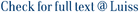Let G be an undirected graph with m edges and n vertices. A spanner of G is a subgraph which preserves approximate distances between all pairs of vertices. An f-vertex fault-tolerant spanner is a subgraph which preserves approximate distances, under the failure of any set of at most f vertices. The contribution of this paper is twofold: we present algorithms for computing fault-tolerant spanners, and propose streaming algorithms for computing spanners in very small internal memory. In particular, we give algorithms for computing f-vertex fault-tolerant (3,2)- and (2,1)-spanners of G with the following bounds: our (3,2)-spanner contains O(f4/3n4/3) edges and can be computed in time Õ(f2m), while our(2, 1)-spanner contains O(fn3/2) edges and can be computed in time . Both algorithms improve significantly on previously known bounds. Assume that the graph G is presented as an input stream of edges, which may appear in any arbitrary order, and that we do not know in advance m and n. We show how to compute efficiently (3, 2)- and (2, 1)-spanners of G, using only very small internal memory and as low access external memory device. Our spanners have asymptotically optimal size and the I/O complexity of our algorithms for computing such spanners is optimal upto apolylogarithmic factor. Our f-vertex fault-tolerant (3, 2)- and (2, 1)-spanners can also be computed efficiently in the same computational model described above.

Computing Graph Spanners in Small Memory: Fault-Tolerance and Streaming / Ausiello, G; Franciosa, P; Italiano, Giuseppe Francesco; Ribichini, A. - In: DISCRETE MATHEMATICS, ALGORITHMS AND APPLICATIONS. - ISSN 1793-8309. - 2:4(2010), pp. 591-605. [10.1142/S1793830910000905]

### Computing Graph Spanners in Small Memory: Fault-Tolerance and Streaming

#### Abstract

Let G be an undirected graph with m edges and n vertices. A spanner of G is a subgraph which preserves approximate distances between all pairs of vertices. An f-vertex fault-tolerant spanner is a subgraph which preserves approximate distances, under the failure of any set of at most f vertices. The contribution of this paper is twofold: we present algorithms for computing fault-tolerant spanners, and propose streaming algorithms for computing spanners in very small internal memory. In particular, we give algorithms for computing f-vertex fault-tolerant (3,2)- and (2,1)-spanners of G with the following bounds: our (3,2)-spanner contains O(f4/3n4/3) edges and can be computed in time Õ(f2m), while our(2, 1)-spanner contains O(fn3/2) edges and can be computed in time . Both algorithms improve significantly on previously known bounds. Assume that the graph G is presented as an input stream of edges, which may appear in any arbitrary order, and that we do not know in advance m and n. We show how to compute efficiently (3, 2)- and (2, 1)-spanners of G, using only very small internal memory and as low access external memory device. Our spanners have asymptotically optimal size and the I/O complexity of our algorithms for computing such spanners is optimal upto apolylogarithmic factor. Our f-vertex fault-tolerant (3, 2)- and (2, 1)-spanners can also be computed efficiently in the same computational model described above.
##### Scheda breve Scheda completa Scheda completa (DC)2010
Graph spanners; external memory; data streaming; fault tolerance
Computing Graph Spanners in Small Memory: Fault-Tolerance and Streaming / Ausiello, G; Franciosa, P; Italiano, Giuseppe Francesco; Ribichini, A. - In: DISCRETE MATHEMATICS, ALGORITHMS AND APPLICATIONS. - ISSN 1793-8309. - 2:4(2010), pp. 591-605. [10.1142/S1793830910000905]
File in questo prodotto:
Non ci sono file associati a questo prodotto.
##### Pubblicazioni consigliate

I documenti in IRIS sono protetti da copyright e tutti i diritti sono riservati, salvo diversa indicazione.

Utilizza questo identificativo per citare o creare un link a questo documento: `https://hdl.handle.net/11385/199799`
##### Citazioni
•8
•8